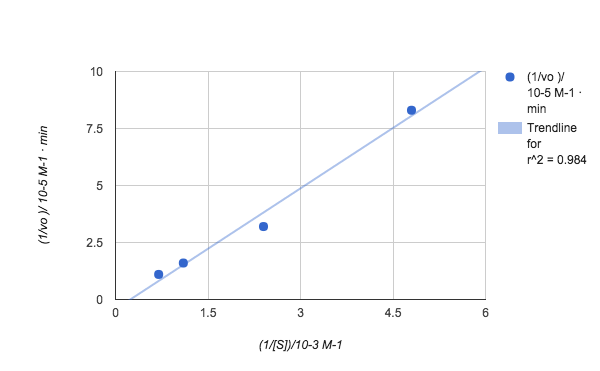# Solutions 7

$$\newcommand{\vecs}{\overset { \rightharpoonup} {\mathbf{#1}} }$$ $$\newcommand{\vecd}{\overset{-\!-\!\rightharpoonup}{\vphantom{a}\smash {#1}}}$$$$\newcommand{\id}{\mathrm{id}}$$ $$\newcommand{\Span}{\mathrm{span}}$$ $$\newcommand{\kernel}{\mathrm{null}\,}$$ $$\newcommand{\range}{\mathrm{range}\,}$$ $$\newcommand{\RealPart}{\mathrm{Re}}$$ $$\newcommand{\ImaginaryPart}{\mathrm{Im}}$$ $$\newcommand{\Argument}{\mathrm{Arg}}$$ $$\newcommand{\norm}{\| #1 \|}$$ $$\newcommand{\inner}{\langle #1, #2 \rangle}$$ $$\newcommand{\Span}{\mathrm{span}}$$ $$\newcommand{\id}{\mathrm{id}}$$ $$\newcommand{\Span}{\mathrm{span}}$$ $$\newcommand{\kernel}{\mathrm{null}\,}$$ $$\newcommand{\range}{\mathrm{range}\,}$$ $$\newcommand{\RealPart}{\mathrm{Re}}$$ $$\newcommand{\ImaginaryPart}{\mathrm{Im}}$$ $$\newcommand{\Argument}{\mathrm{Arg}}$$ $$\newcommand{\norm}{\| #1 \|}$$ $$\newcommand{\inner}{\langle #1, #2 \rangle}$$ $$\newcommand{\Span}{\mathrm{span}}$$

## S1

Fill in the blanks.

Many enzymes require a nonprotein cofactor to assist them in their reaction. In this case, the protein portion of the enzyme, called an ___apoenzyme___ , combines with the cofactor to form the whole enzyme or __haloenzyme__ . Some cofactors are ions such as Ca++, Mg++, and K+; other cofactors are organic molecules called __coenzymes__ which serve as carriers for chemical groups or electrons. Anything that an enzyme normally combines with is called a __substrate__ .

## S2

Enzymes are substances present in the cell in small amounts that function to speed up or catalyze chemical reactions. On the surface of the enzyme is usually a small crevice that functions as an active site or catalytic site to which one or two specific substrates are able to bind. Anything that an enzyme normally combines with is called a substrate. The binding of the substrate to the enzyme causes the flexible enzyme to change its shape slightly through a process called induced fit to form a temporal intermediate called an enzyme-substrate complex.

Enzymes speed up the rate of chemical reactions because they lower the energy of activation, the energy that must be supplied in order for molecules to react with one another. Enzymes lower the energy of activation by forming an enzyme-substrate complex allowing products of the enzyme reaction to be formed and released

## S3

State how the following will affect the rate of an enzyme reaction.

1. increasing temperature

Ans: Each enzyme has an optimum temperature at which it works best. A higher temperature generally results in an increase in enzyme activity. As the temperature increases, molecular motion increases resulting in more molecular collisions. If, however, the temperature rises above a certain point, the heat will denature the enzyme, causing it to lose its three-dimensional functional shape by denaturing its hydrogen bonds.

2. decreasing temperature

Ans: Cold temperature slows down enzyme activity by decreasing molecular motion.

3. PH

Ans: Each enzyme has an optimal pH that helps maintain its three-dimensional shape. Changes in pH may denature enzymes by altering the enzyme's charge. This alters the ionic bonds of the enzyme that contribute to its functional shape.

4. salt concentration

Ans: Each enzyme has an optimal salt concentration. Changes in the salt concentration may also denature enzymes.

## S4

$k_{3}[Br^{*}][H_{2}]$

## S5

We already know the turnover number ($$k_{cat}$$). The amount of time necessary to cleave the substrate is the reciprocal of the turnover rate.

$t= \dfrac{1}{k} = \dfrac{1}{30,000 \;s^{-1}} = 3.33 \times 10^{-5} s$

## S6

1. Find the initial velocity.

$$v_0 = \dfrac{25\ \mu M}{0.75\ min} \]$$ v_0 = 33.3\ \mu M/min \]

2. Use v0, [S], and KM to solve for Vmax.

$$v_0 = \dfrac{V_{max} [S]}{K_M + [S]} \]$$ V_{max} = v_0 \left ( \dfrac{K_M}{[S]} + 1 \right ) \]

$$V_{max} = 33.3\ \mu M/min \left ( \dfrac{1.5\ mM}{0.25\ M} \times \dfrac{M}{1000\ mM} + 1 \right ) \]$$ V_{max} = 33.3\ \mu M/min \]

Notice that ν0 = Vmax. Since [S] >>KM, the reaction will continue with a velocity of Vmax for the remainder of the two minutes.

3. Predict [P] after 2.0 minutes at the rate Vmax.

$$[P] = V_{max} \times t \]$$ [P] = 33.3\ \mu M/min \times 2.0\ min \]

 [P] = 66.6\ \mu M \]

## S7

 (1/[S])/10^3 M-1 (1/vo )/ 10^5 M-1 · min 4.8 8.3 2.4 3.2 1.1 1.6 0.7 1.1

Make the Lineweaver-Burk PlotThe linear equation for the graph above is: y=176x+4.23X104 To solve for Vmax use: Intercept= 1/Vmax Vmax = 1/(4.23X104) = 2.36 X 10-5 To solve for Km use Slope= Km/Vmax Km=Slope x Vmax=(176)( 2.36 X 10-5) = 4.2 X 10-3 M To solve for K2 use K2 = Vmax/ [E]0 = (2.36 X 10-5) / (5.0 X 10-6​ M)= 4.72 min-1

Solutions 7 is shared under a not declared license and was authored, remixed, and/or curated by LibreTexts.